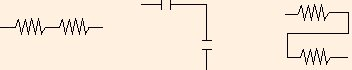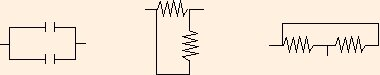### Capacitance

1. The capacitance of a capacitor for storing charge is the ratio of the charge stored to the potential difference "across" the capacitor:
C ≡ Q / ΔV.

Capacitance is measured in Farads (1 F ≡ 1 C / V). Note that ε also has units of F / m.

Capacitance depends only on the geometry of the capacitor and the permittivity. To compute the capacitance, use Gauss' law to compute E and integrate to get ΔV.

2. Two electrical components are said to be in series if they are connected directly together at one end of each, and nothing else is connected there:Any charge that enters one side of a series configuration must leave the other side.
Two capacitors in series have the same charge, so if we replace them with an equivalent capacitor, we must have
Qeq = Q1 = Q2

ΔVeq = ΔV1 + ΔV2

or
1 / Ceq = 1 / C1 + 1 / C2
3. Two electrical components are said to be in parallel if they are connected directly together at both ends, and nothing else is connected between them:Since conductors are equipotentials, components in a parallel configuration must be at the same potential where they are connected.
Two capacitors in parallel have the same ΔV ("voltage drop"), so if we replace them with an equivalent capacitor, we must have
ΔVeq = ΔV1 = ΔV2

Qeq = Q1 + Q2

or
Ceq = C1 + C2
4. The work required to charge a capacitor is
∫ ΔV dq
= Q2 / (2 C)

= C ΔV2 / 2.

If we think of this energy as being stored in the electric field within the capacitor, we can compute the energy density of the electric field to be
uE = ε E2 / 2.
(This can be shown using a parallel plate capacitor:
C ΔV2 / 2 / (area * distance) = ε ΔV2 / (2 distance2)
= ε (E * distance)2 / (2 distance2)
but it is true in general.)
5. This applet will allow you to practice analysis of some simple capacitor networks (for the moment, ignore the questions involving resistors).
6. Define the dipole moment of two oppositely charged particles (± q), separated by a distance r, as
p ≡ q r,
where r points from -q to +q. Then the torque experienced by the dipole in an electric field is
τ = p ⊗ E
and the potential energy of the dipole is
U = - p ⋅ E.

 (prev) (top) (next)